## Heating of Aluminium Plate with Hole

### Experiment number : 2127

• #### Goal of experiment

We have an aluminium plate with a round hole in the middle. The goal of this experiment is to show what happens with the hole if we heat it.

• #### Theory

Most substances increase their volume when heated. If we heat an aluminium plate above a candle, the plate expands. This phenomenon is called thermal expansion.

If we heat a rod with initial length l0 and initial temperature t0 to temperature t, the length of the rod increases to l. The length l after heating is calculated from the relationship

$l = l_{0}(1+αΔt),$

where α is the coefficient of thermal expansion. This relationship applies to objects with one dimension considerably larger than the other two.

The hole in the middle of the plate has a diameter d0 at temperature t0. If we heat this plate to a temperature t, the diameter of the hole increases. We can calculate the diameter d of the hole after heating from the relationship

$d = d_{0}(1+αΔt).$

When heating the aluminium plate, a ball placed in the hole is also heated. The ball touches the plate with only a very small area. Therefore, the expansion of the ball can be neglected in comparison with the expansion of the plate.

The task supporting this experiment can be found here: Change in the Metal Plate's Size during Heating.

• #### Tools

• metal plate with round hole (we used a duralumin/aluminium plate)

• holder

• ball with a slightly larger diameter than the diameter of the hole in the plate before heating

• candle

• lighter

• soft object to catch the falling ball (we used a piece of cloth)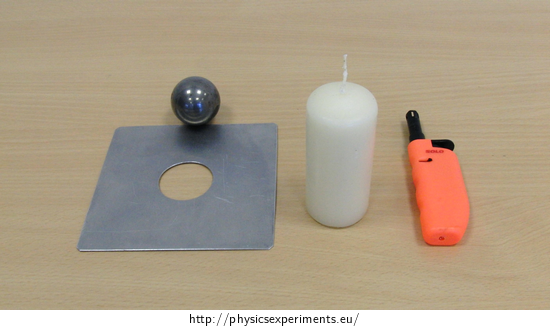• #### Procedure

1. Fix the metal plate to the holder so that it is parallel to the table.

2. Place a ball in the hole so that it does not fall through the hole.

3. Put a candle below the plate and light it.

4. The plate is heated, the diameter of the hole increases and the ball is dropped.

5. Remove the candle. The plate temperature decreases, so does the hole diameter and the ball does not fit through the hole.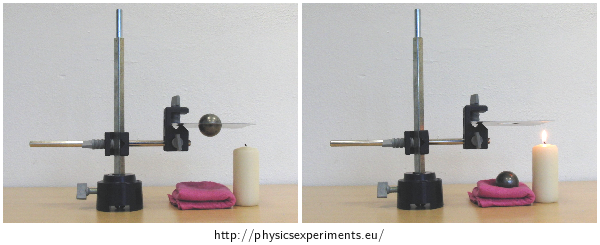• #### Sample result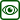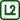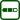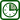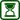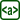×Original source: Inspirováno: Epstein, L.C., Hewitt, P., C.: Thonking Physics. Insight Press, 1981